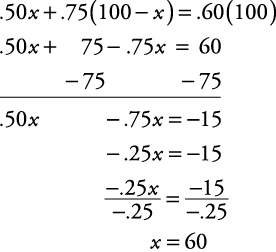## Mixture Problems

Here are some examples for solving mixture problems.

##### Example 1

Coffee worth \$1.05 per pound is mixed with coffee worth 85¢ per pound to obtain 20 pounds of a mixture worth 90¢ per pound. How many pounds of each type are used?

First, circle what you are trying to find— how many pounds of each type. Now, let the number of pounds of \$1.05 coffee be denoted as x. Therefore, the number of pounds of 85¢‐per‐pound coffee must be the remainder of the twenty pounds, or 20 – x. Now, make a chart for the cost of each type and the total cost.

cost per lb.

x

amount in lbs.

=

total cost of each

\$1.05 coffee

\$1.05

x

\$1.05 x

\$ .85 coffee

\$ .85

20–x

\$ .85(20–x)

mixture

\$ .90

20

\$ .90(20)

Now, set up the equation.Therefore, five pounds of coffee worth \$1.05 per pound are used. And 20 – x , or 20 – 5, or fifteen pounds of 85¢‐per‐pound coffee are used.

##### Example 2

Solution A is 50% hydrochloric acid, while solution B is 75% hydrochloric acid. How many liters of each solution should be used to make 100 liters of a solution which is 60% hydrochloric acid?

First, circle what you're trying to find— liters of solutions A and B. Now, let x stand for the number of liters of solution A. Therefore, the number of liters of solution B must be the remainder of the 100 liters, or 100 – x. Next, make the following chart.

% of acid

liters

concentration of acid

solution A

50%

x

.50 x

solution B

75%

100–x

.75(100–x)

new solution

60%

100

.60(100)

Now, set up the equation.Therefore, using the chart, 60 liters of solution A and 40 liters of solution B are used.